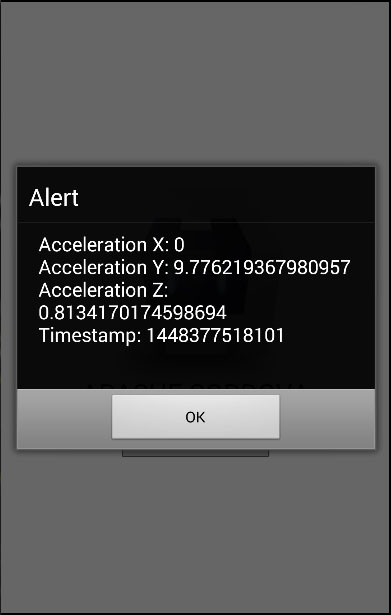# Cordova - Accelerometer

The Accelerometer plugin is also called the device-motion. It is used to track device motion in three dimensions.

## Step 1 - Install Accelerometer Plugin

We will install this plugin by using cordova-CLI. Type the following code in the command prompt window.

```C:\Users\username\Desktop\CordovaProject>cordova plugin add cordova-plugindevice-motion
```

## Step 2 - Add Buttons

In this step, we will add two buttons in the index.html file. One will be used for getting the current acceleration and the other will watch for the acceleration changes.

```<button id = "getAcceleration">GET ACCELERATION</button>
<button id = "watchAcceleration">WATCH ACCELERATION</button>
```

## Step 3 - Add Event Listeners

Let us now add event listeners for our buttons to onDeviceReady function inside index.js.

```document.getElementById("getAcceleration").addEventListener("click", getAcceleration);
"click", watchAcceleration);
```

## Step 4 - Creating Functions

Now, we will create two functions. The first function will be used to get the current acceleration and the second function will watch the acceleration and the information about the acceleration will be triggered every three seconds. We will also add the clearWatch function wrapped by the setTimeout function to stop watching acceleration after the specified time frame. The frequency parameter is used to trigger the callback function every three seconds.

```function getAcceleration() {
navigator.accelerometer.getCurrentAcceleration(
accelerometerSuccess, accelerometerError);

function accelerometerSuccess(acceleration) {
alert('Acceleration X: ' + acceleration.x + '\n' +
'Acceleration Y: ' + acceleration.y + '\n' +
'Acceleration Z: ' + acceleration.z + '\n' +
'Timestamp: '      + acceleration.timestamp + '\n');
};

function accelerometerError() {
};
}

function watchAcceleration() {
var accelerometerOptions = {
frequency: 3000
}
var watchID = navigator.accelerometer.watchAcceleration(
accelerometerSuccess, accelerometerError, accelerometerOptions);

function accelerometerSuccess(acceleration) {
alert('Acceleration X: ' + acceleration.x + '\n' +
'Acceleration Y: ' + acceleration.y + '\n' +
'Acceleration Z: ' + acceleration.z + '\n' +
'Timestamp: '      + acceleration.timestamp + '\n');

setTimeout(function() {
navigator.accelerometer.clearWatch(watchID);
}, 10000);
};

function accelerometerError() {